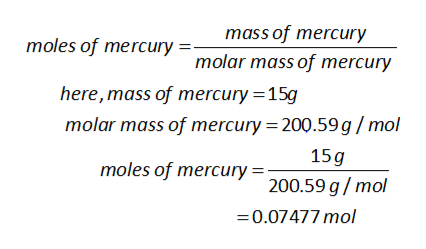# Calculate the heat energy released when 15.0 g of liquid mercury at 25.00 °C is converted to solid mercury at its melting point. Constants for mercury at 1 atmheat capacity of Hg(l)28.0 J/(mol⋅K)melting point234.32 Kenthalpy of fusion2.29 kJ/mol  qp= _____________ kJ

Question
100 views
Calculate the heat energy released when 15.0 g of liquid mercury at 25.00 °C is converted to solid mercury at its melting point.

Constants for mercury at 1 atm
heat capacity of Hg(l) 28.0 J/(mol⋅K)
melting point 234.32 K
enthalpy of fusion 2.29 kJ/mol

qp= _____________ kJ
check_circle

Step 1

given data as follows:

Step 2

To calculate the number of moles is mass of substance divided by molar mass of substance.help_outlineImage Transcriptionclosemass of mercury molar mass of mercury moles of mercury here, mass of mercury 15g molar mass of mercury 200.59g /mol 15g 200.59 g/mol moles of mercury 0.07477mol fullscreen
Step 3

To determine the heat released on cooling liquid mercury fr...

### Want to see the full answer?

See Solution

#### Want to see this answer and more?

Solutions are written by subject experts who are available 24/7. Questions are typically answered within 1 hour.*

See Solution
*Response times may vary by subject and question.
Tagged in

### Chemistry